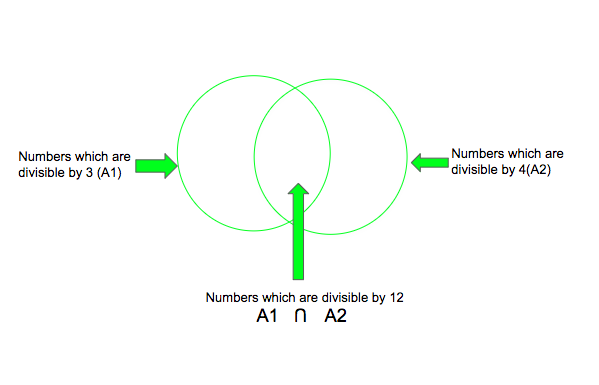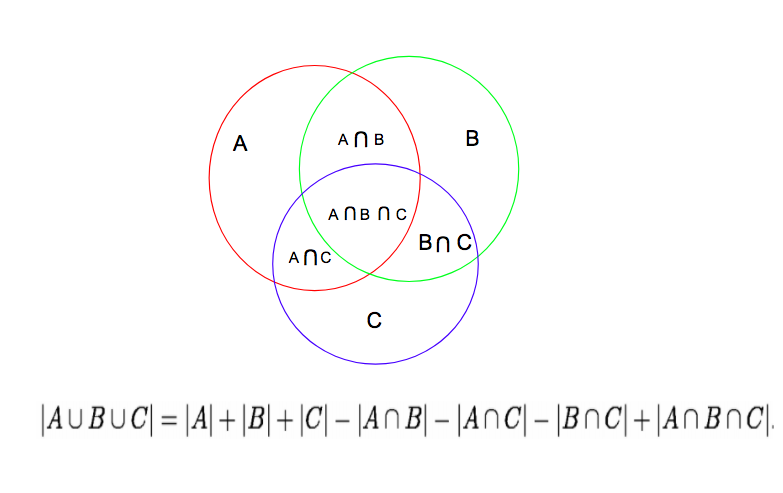Skip to content
Related Articles
Inclusion Exclusion principle and programming applications

Sum Rule – If a task can be done in one of n1 ways or one of n2 ways, where none of the set of n1 ways is the same as any of the set of n2 ways, then there are n1 + n2 ways to do the task. The sum-rule mentioned above states that if there are multiple sets of ways of doing a task, there shouldn’t be any way that is common between two sets of ways because if there is, it would be counted twice and the enumeration would be wrong.

The principle of inclusion-exclusion says that in order to count only unique ways of doing a task, we must add the number of ways to do it in one way and the number of ways to do it in another and then subtract the number of ways to do the task that are common to both sets of ways.

The principle of inclusion-exclusion is also known as the subtraction principle. For two sets of ways Aiand  A2, the enumeration would like-
| A1 ∪ A2  |= |A1 |+ | A2| – |A1 ∩ A2|

Below are some examples to explain the application of inclusion-exclusion principle:

Example 1: How many binary strings of length 8 either start with a ‘1’ bit or end with two bits ’00’?

Solution: If the string starts with one, there are 7 characters left which can be filled in 27 = 128 ways.
If the string ends with ’00’ then 6 characters can be filled in 2 6 = 64 ways.

Now if we add the above sets of ways and conclude that it is the final answer, then it would be wrong. This is because there are strings with start with ‘1’ and end with ’00’ both, and since they satisfy both criteria they are counted twice.
So we need to subtract such strings to get a correct count.
Strings that start with ‘1’ and end with ’00’ have five characters that can be filled in 25=32 ways.
So by the inclusion-exclusion principle we get- Total strings = 128 + 64 – 32 = 160

Example 2: How many numbers between 1 and 1000, including both, are divisible by 3 or 4?
Solution: Number of numbers divisible by 3 =. Number of numbers divisible by 4 =.Number of numbers divisible by 3 and 4 =Therefore, number of numbers divisible by 3 or 4 == 333 + 250 – 83 = 500

Implementation

Problem 1: How many numbers between 1 and 1000, including both, are divisible by 3 or 4?
The Approach will be the one discussed above, we add the number of numbers that are divisible by 3 and 4 and subtract the numbers which are divisible by 12.## C++

 `// CPP program to count the``// number of numbers between``// 1 and 1000, including both,``// that are divisible by 3 or 4``#include ``using` `namespace` `std;` `// function to count the divisors``int` `countDivisors(``int` `N, ``int` `a, ``int` `b)``{``    ``// Counts of numbers``    ``// divisible by a and b``    ``int` `count1 = N / a;``    ``int` `count2 = N / b;` `    ``// inclusion-exclusion``    ``// principle applied``    ``int` `count3 = (N / (a * b));` `    ``return` `count1 + count2 - count3;``}` `// Driver Code``int` `main()``{``    ``int` `N = 1000, a = 3, b = 4;``    ``cout << countDivisors(N, a, b);``    ``return` `0;``}`

## Java

 `// Java program to count the``// number of numbers between``// 1 and 1000, including both,``// that are divisible by 3 or 4``import` `java.io.*;` `class` `GFG {` `    ``// function to count the divisors``    ``public` `static` `int` `countDivisors(``int` `N, ``int` `a, ``int` `b)``    ``{``        ``// Counts of numbers``        ``// divisible by a and b``        ``int` `count1 = N / a;``        ``int` `count2 = N / b;` `        ``// inclusion-exclusion``        ``// principle applied``        ``int` `count3 = (N / (a * b));` `        ``return` `count1 + count2 - count3;``    ``}` `    ``// Driver Code``    ``public` `static` `void` `main(String[] args)``    ``{``        ``int` `N = ``1000``, a = ``3``, b = ``4``;``        ``System.out.println(countDivisors(N, a, b));``    ``}``}` `// This code is contributed by m_kit`

## Python3

 `# Python3 program to count the``# number of numbers between``# 1 and 1000, including both,``# that are divisible by 3 or 4` `# function to count the divisors`  `def` `countDivisors(N, a, b):` `    ``# Counts of numbers``    ``# divisible by a and b``    ``count1 ``=` `N ``/``/` `a``    ``count2 ``=` `N ``/``/` `b` `    ``# inclusion-exclusion``    ``# principle applied``    ``count3 ``=` `(N ``/``/` `(a ``*` `b))` `    ``return` `count1 ``+` `count2 ``-` `count3`  `# Driver Code``N ``=` `1000``a ``=` `3``b ``=` `4``print``(countDivisors(N, a, b))` `# This code is contributed by shubhamsingh10`

## C#

 `// C# program to count the``// number of numbers between``// 1 and 1000, including both,``// that are divisible by 3 or 4``using` `System;` `class` `GFG {` `    ``// function to count``    ``// the divisors``    ``public` `static` `int` `countDivisors(``int` `N, ``int` `a, ``int` `b)``    ``{``        ``// Counts of numbers``        ``// divisible by a and b``        ``int` `count1 = N / a;``        ``int` `count2 = N / b;` `        ``// inclusion-exclusion``        ``// principle applied``        ``int` `count3 = (N / (a * b));` `        ``return` `count1 + count2 - count3;``    ``}` `    ``// Driver Code``    ``static` `public` `void` `Main()``    ``{``        ``int` `N = 1000, a = 3, b = 4;``        ``Console.WriteLine(countDivisors(N, a, b));``    ``}``}` `// This code is contributed by aj_36`

## PHP

 ``

## Javascript

 ``

Output :

`500`

Problem 2: Given N prime numbers and a number M, find out how many numbers from 1 to M are divisible by any of the N given prime numbers.

Examples :

```Input: N numbers = {2, 3, 5, 7}   M = 100
Output: 78

Input: N numbers = {2, 5, 7, 11}   M = 200
Output: 137```

The approach for this problem will be to generate all the possible combinations of numbers using N prime numbers using power set in 2N. For each of the given prime numbers Pi among N, it has M/Pi multiples. Suppose M=10, and we are given with 3 prime numbers(2, 3, 5), then the total count of multiples when we do 10/2 + 10/3 + 10/5 is 11. Since we are counting 6 and 10 twice, the count of multiples in range 1-M comes 11. Using inclusion-exclusion principle, we can get the correct number of multiples. The inclusion-exclusion principle for three terms can be described as:Similarly, for every N numbers, we can easily find the total number of multiples in range 1 to M by applying the formula for an intersection of N numbers. The numbers that are formed by multiplication of an odd number of prime numbers will be added and the numbers formed by multiplication of even numbers will thus be subtracted to get the total number of multiples in the range 1 to M.

Using power set we can easily get all the combination of numbers formed by the given prime numbers. To know if the number is formed by multiplication of odd or even numbers, simply count the number of set bits in all the possible combinations (1-1<<N).
Using power sets and adding the numbers created by combinations of odd and even prime numbers we get 123 and 45 respectively. Using inclusion-exclusion principle we get the number of numbers in range 1-M that is divided by any one of N prime numbers is (odd combinations-even combinations) = (123-45) = 78.

Below is the implementation of the above idea:

## C++

 `// CPP program to count the``// number of numbers in range``// 1-M that are divisible by``// given N prime numbers``#include ``using` `namespace` `std;` `// function to count the number``// of numbers in range 1-M that``// are divisible by given N``// prime numbers``int` `count(``int` `a[], ``int` `m, ``int` `n)``{``    ``int` `odd = 0, even = 0;``    ``int` `counter, i, j, p = 1;``    ``int` `pow_set_size = (1 << n);` `    ``// Run from counter 000..0 to 111..1``    ``for` `(counter = 1; counter < pow_set_size; counter++) {``        ``p = 1;``        ``for` `(j = 0; j < n; j++) {` `            ``// Check if jth bit in the``            ``// counter is set If set``            ``// then print jth element from set``            ``if` `(counter & (1 << j)) {``                ``p *= a[j];``            ``}``        ``}` `        ``// if set bits is odd, then add to``        ``// the number of multiples``        ``if` `(__builtin_popcount(counter) & 1)``            ``odd += (m / p);``        ``else``            ``even += (m / p);``    ``}` `    ``return` `odd - even;``}` `// Driver Code``int` `main()``{``    ``int` `a[] = { 2, 3, 5, 7 };``    ``int` `m = 100;``    ``int` `n = ``sizeof``(a) / ``sizeof``(a);``    ``cout << count(a, m, n);``    ``return` `0;``}`

## Java

 `// Java program to count the``// number of numbers in range``// 1-M that are divisible by``// given N prime numbers` `import` `java.io.*;` `class` `GFG {``    ``// function to count the number``    ``// of numbers in range 1-M that``    ``// are divisible by given N``    ``// prime numbers``    ``public` `static` `int` `count(``int` `a[], ``int` `m, ``int` `n)``    ``{``        ``int` `odd = ``0``;``        ``int` `even = ``0``;``        ``int` `counter = ``0``;``        ``int` `i = ``0``;``        ``int` `j = ``0``;``        ``int` `p = ``1``;``        ``int` `pow_set_size = (``1` `<< n);` `        ``// Run from counter 000..0 to 111..1``        ``for` `(counter = ``1``; counter < pow_set_size;``             ``counter++) {``            ``p = ``1``;``            ``for` `(j = ``0``; j < n; j++) {``                ``// Check if jth bit in the``                ``// counter is set If set``                ``// then pront jth element from set``                ``if` `((counter & (``1` `<< j)) > ``0``) {``                    ``p *= a[j];``                ``}``            ``}` `            ``// if set bits is odd, then add to``            ``// the number of multiples``            ``if` `(Integer.bitCount(counter) % ``2` `== ``1``) {``                ``odd += (m / p);``            ``}``            ``else` `{``                ``even += (m / p);``            ``}``        ``}` `        ``return` `odd - even;``    ``}` `    ``// Driver Code``    ``public` `static` `void` `main(String[] args)``    ``{``        ``int` `a[] = { ``2``, ``3``, ``5``, ``7` `};``        ``int` `m = ``100``;``        ``int` `n = a.length;``        ``System.out.println(count(a, m, n));``    ``}``}`

## Python3

 `# Python3 program to count the``# number of numbers in range``# 1-M that are divisible by``# given N prime numbers`  `def` `bitsoncount(x):``    ``return` `bin``(x).count(``'1'``)` `# function to count the number``# of numbers in range 1-M that``# are divisible by given N``# prime numbers`  `def` `count(a, m, n):` `    ``odd ``=` `0``    ``even ``=` `0``    ``p ``=` `1``    ``pow_set_size ``=` `1` `<< n` `    ``# Run from counter 000..0 to 111..1``    ``for` `counter ``in` `range``(``1``, pow_set_size):` `        ``p ``=` `1``        ``for` `j ``in` `range``(n):` `            ``# Check if jth bit in the``            ``# counter is set If set``            ``# then pront jth element from set``            ``if` `(counter & (``1` `<< j)):` `                ``p ``*``=` `a[j]` `        ``# if set bits is odd, then add to``        ``# the number of multiples``        ``if` `(bitsoncount(counter) & ``1``):``            ``odd ``+``=` `(m ``/``/` `p)``        ``else``:` `            ``even ``+``=` `(m ``/``/` `p)` `    ``return` `odd ``-` `even`  `# Driver Code``a ``=` `[``2``, ``3``, ``5``, ``7``]``m ``=` `100``n ``=` `len``(a)``print``(count(a, m, n))` `# This code is contributed by shivanisinghss2110`

## Javascript

 ``

Output:

`78`

Time Complexity: O(2N*N)

Attention reader! Don’t stop learning now. Get hold of all the important DSA concepts with the DSA Self Paced Course at a student-friendly price and become industry ready.  Get hold of all the important mathematical concepts for competitive programming with the Essential Maths for CP Course at a student-friendly price.

In case you wish to attend live classes with industry experts, please refer Geeks Classes Live

My Personal Notes arrow_drop_up